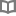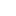不出門也能開心玩的33個居家親子遊戲 - 近藤理恵 - BOOKWALKER中文電子書

不出門也能開心玩的33個居家親子遊戲

近藤理恵／文．插畫

插畫家，出生於東京。於武藏野美術大學、研究所學習日本畫。兒子小時候很頑皮、常常發燒，為了保有在家工作的時間，每天都要絞盡腦汁想出各種點子。 這本書就是源自於當時跟兒子相處的時光，以及目前擔任美術創作班老師接觸孩童們，再加上多方結識的媽媽友、保姆。

• 放入購物車
•試閱

• 放入購物車
•無試閱

• 放入購物車
•試閱

• 放入購物車
•試閱

• 放入購物車
•試閱

• 放入購物車
•試閱

• 放入購物車
•試閱

• 放入購物車
•試閱

• 放入購物車
•試閱

• 放入購物車
•試閱

• 放入購物車
•試閱

• 放入購物車
•試閱

• 放入購物車
•無試閱

• 放入購物車
•試閱

• 放入購物車
•試閱

• 放入購物車
•試閱

• 放入購物車
•試閱

• 放入購物車
•試閱

• 放入購物車
•試閱

• 放入購物車
•試閱

• 放入購物車
•試閱

• 放入購物車
•試閱

• 放入購物車
•試閱

• 放入購物車
•試閱

• 放入購物車
•試閱

• 放入購物車
•試閱

• 放入購物車
•試閱

• 放入購物車
•試閱

• 放入購物車
•試閱

• 放入購物車
•試閱

• 放入購物車
•試閱

• 放入購物車
•試閱

• 放入購物車
•試閱

• 放入購物車
•試閱

• 放入購物車
•試閱

• 放入購物車
•試閱

• 放入購物車
•試閱

• 放入購物車
•試閱

• 放入購物車
•試閱

• 放入購物車
•試閱